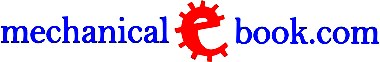Custom Search
 A B C D E F G H I J K L M N O P Q R S T U V W X Y Z
 Electrostatics II The Van de Graaff generator (Fig.1) makes use of the possibility of spraying an electric charge from sharp points of a conductor. The charge is applied to a belt conveyor made of an insulating material, which conveys the charge into the interior of a spherical conductor of large radius, where the charge is collected by a comb of pointed electrodes. More and more electricity is collected in this way from the traveling belt and is accumulated at the surface of the large sphere, which thus acquires a very high charge. Since ancient history it is known that some materials attract light particles after rubbing. Electrostatic phenomena arise from the forces that electric charges carry out on each other. Such forces are described by Coulomb's law. Even though electrostatically induced forces seem to be rather weak, the electrostatic force between e.g., an electron and a proton, that together make up a hydrogen atom, is about 40 orders of magnitute stronger than the gravitational force acting between them. The power output from this electrostatic generator is not very large, for the charge accumulated by this method cannot sustain a current of any significant magnitude. On the other hand, very high voltages can be obtained of the order of some millions of volts. The voltage can be further increased by installing the generator in an enclosed space in which the air pressure is increased above the normal atmospheric pressure, so that the spark-over voltage to earthed components is increased. The amount of electric charge that can be stored up in a body is called the capacity of that body. A condenser or capacitor is a device specifically intended to store up an electric charge. Its capacity is determined mainly by the action of electrostatic induction. It has essentially of two conducting surfaces which are insulated from each other (Fig.2a). In the case of a variable condenser the area (F) of these surfaces and/or their distance apart (d1, d2) can be varied.. Obviously, the quantity of electricity that can be stored up by induction will be greater according as F is larger and the gap d between the condenser plates is smaller. The capacity of a plate condenser is therefore proportional to F and inversely proportional to d. High capacity condensers are composed of plates consisting of rolled-up thin metal foils separated by sheets of paper as the insulating medium. The capacity of a condenser may be compared with the cubic capacity of a tank, which depends on the area of the bottom and on the height (Fig.2b). If a small ball pendulum is attached to a conductor and the latter is charged with electricity, the pendulum will acquire a deflection due to electrical repulsion which is proportional to the magnitude of the charge (Fig.3a). there is an analogy with the pressure of water in a tank, which pressure can be measured by means of a mercury manometer or pressure gauge (Fig.3b). The pressure of the water corresponds to the electric potential or voltage (the unit of measurement being the volt.) The voltage (U) is associated with the electric charge Q and the capacity C of a conductor (measured in farads) by the following relation: U = Q / C. In the space which surrounds an electrically charged body an electric potential occurs which is proportional to the charge Q and inversely proportional to the distance r from the center of the body (U = Q / r). The electrical condition produced in a space by the presence of electrically charged bodies is called an electric field (Fig.4) points which all have the same potential (voltage) are located on equipotential surfaces. Forces always act in the direction of the potential gradient. The electric force which is exerted upon a charge of unit magnitude in an electric field is called the field strength. It is always directed perpendicularly to the equipotential surfaces. The lines of force in an electric field represent the direction of the force at any point on their length. The properties of an electric field can be described in terms of equipotential surfaces and lines of force. The lines of force are conceived as emerging from positive charges and disappearing into negative charges. o DISCLAIMER         o CONTACT US